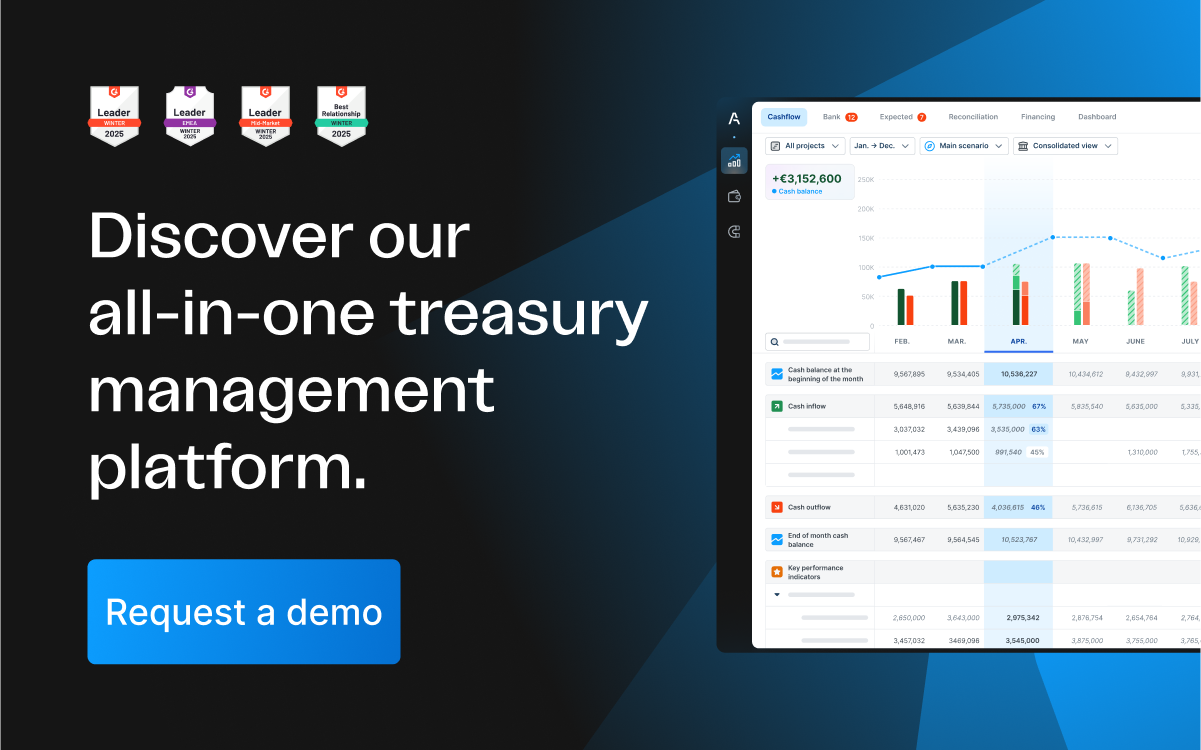# What is meant by future cash flow and how can you predict it?The future cash flow indicates how high the expected income and expenditure of a company will be. With its help, it is possible to estimate how the cash situation of a company will develop. We show you here why this is important and how to determine the value of future cash flows at the current time.

## What is future cash flow?

The future cash flow is all income and expenditure that a company expects in a future period. A forecasting period of several days, several months or even several years can be assumed.In order to best estimate future cash flow, one needs to know all the influences on income and expenses, as well as the economic interrelationships between different factors. This can be a very demanding task, but it is worth the effort, because with an accurate estimate of future cash flows you can optimally plan your operational business.

Key questions that can be asked when estimating future expenditure and income are, for example:

• How will customer demand develop?
• Are investments planned? If so, what expenses will they incur and what income will they generate?
• Are delivery problems expected? If so, how will these affect production and ultimately the income from sales?
• How are the prices for the required raw materials and materials developing and what impact does this have on expenditure?## Why is future cash flow important?

Estimating future cash flow is important because this forecast shows how much cash a company is likely to have available in the coming period. Important insights can then be derived from this. For example, it is easier to recognise when a cash shortage is imminent and to take precautions in time.

One also recognises when one will have sufficient surpluses available to make investments. In this way, favourable times can be estimated and one can avoid having to take out a loan for smaller investments, which would only burden liquidity.

## How to calculate future cash flow?

The future cash flow is calculated in the same way as the past cash flows. All expenses are deducted from the income within a certain period of time.

## Future cash flow formula

Future cash flow = Future revenues - future expenses

When calculating revenues and expenses, always make sure that they refer to the exact same period, e.g. to a month or a year, otherwise large discrepancies will arise that will lead to an inaccurate future cash flow.

Revenues and expenses include all receipts and payments on the business accounts that are related to the operating business, for example:

Revenues

• Revenue from sales
• Income from financial investments
• Grants
• Tax refunds
• Other revenues
• Expenses

Salary payments and wages

• Inventory
• Expenses for marketing
• General expenses (electricity, bin collection, etc.)
• Fees for software subscriptions and licenses
• Investments
• Tax payments

## Present value of future cash flows: Formula and Example

Calculating the value of future cash flows at the current time is called discounted cash flow (DCF). Here, the expected future cash flows are discounted with a certain interest rate and the present value of the cash flows at the current time is obtained.

This calculation is important if you want to estimate how much the future income is worth at the present time. It makes it easier to judge whether an investment is worthwhile today or not, because you then know how much this investment will generate in cash flow in the future.

The discounted cash flow is calculated with the following formula:

*Discounted cash flow (DCF) = (CF(1) / (1+r)^1) + (CF(2) / (1+r)^2) + (CF(3) / (1+r)^3) + (...)

• (CF(n) / (1+r)^n)*

CF(n) is the cash flow in year n, r is the discounting rate and n is the number of years over which the DCF is calculated.

## Example for discounting future cash flows

A company generates a free cash flow of £200,000 per year with a growth rate of 5%. We first calculate the future cash flows for the next 3 years:

CF1 = £200,000 CF2 = £200,000 + (£200,000 x 0.05) = £210,000 CF3 = £210,000 + (£210,000 x 0.05) = £220,500

Now we assume a discounting rate of 15% per year, which takes into account the decline in value due to inflation and investment risks. We therefore calculate the DCF with:

DCF = £200,000/(1+0.15)^1 + £210,000/(1+0.15)^2 + £220,500/(1+0.15)^3 DCF = £173,913 + £158,790 + £144,982 = £477,685

The future cash flows expected over the next three years are therefore worth £477,685 at the current time.